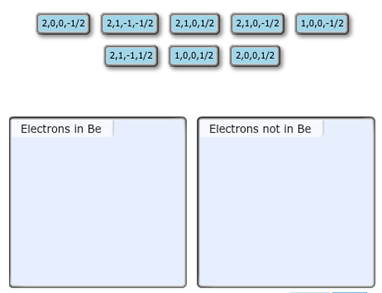# Problem: Each set is ordered(n,l,ml,ms). Identify the sets of quantum numbers that describe all the electrons in the ground state of a neutral beryllium atom, Be. Each set is ordered(n,l,ml,ms). Choose the appropriate items to their respective bins.

🤓 Based on our data, we think this question is relevant for Professor Auzanneau's class at University of Guelph.

###### Problem Details

Each set is ordered(n,l,ml,ms). Identify the sets of quantum numbers that describe all the electrons in the ground state of a neutral beryllium atom, Be. Each set is ordered(n,l,ml,ms). Choose the appropriate items to their respective bins.What scientific concept do you need to know in order to solve this problem?

Our tutors have indicated that to solve this problem you will need to apply the Quantum Numbers concept. If you need more Quantum Numbers practice, you can also practice Quantum Numbers practice problems.

What is the difficulty of this problem?

Our tutors rated the difficulty ofEach set is ordered(n,l,ml,ms). Identify the sets of quantum...as high difficulty.

How long does this problem take to solve?

Our expert Chemistry tutor, Dasha took 5 minutes and 8 seconds to solve this problem. You can follow their steps in the video explanation above.

What professor is this problem relevant for?

Based on our data, we think this problem is relevant for Professor Auzanneau's class at University of Guelph.